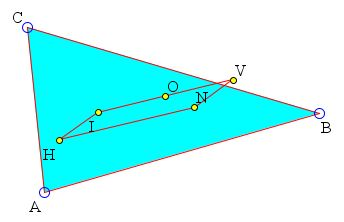A Parallelogram in Triangle

The applet below attempts to illustrate a problem from Mathematics Magazine, Vol. 26, No. 5 (May - Jun., 1953), p. 283 which in the accepted terminology asserts that the four points associated with a triangle - the incenter, the orthocenter, the Nagel and Bevan points - form a parallelogram.BTW, the only browser that runs Java is Forefox. Please declare the site cut-the-knot.org as trusted in Java setup
 What if applet does not run?

ExplanationTo remind, the Nagel point N is the point of concurrency of the three cevians joining the vertices of a triangle to the points of tangency of the side with the corresponding excircle. The Bevan point V is the circumcenter of the excentral triangle and is also the point of concurrency of the perpendiculars from the excenters of a triangle to the corresponding sides.A solution to the problem by L. Bankoff makes use of the known properties of triangle centers. We'll use the conventional notations, H for the orthocenter, I for the incenter, O for the circumcenter, G for the centroid, F for the point, S for the Spieker center, and U for the midpoint of HN.

Bankoff observes that IO is parallel to HN and IO = HN/2 [Johnson, p. 226]. In addition, O is the midpoint of IV. This implies HN = IV which concludes the proof.

Bankoff further notes additional properties of the configuration:

 Furthermore, S is the midpoint IN (which is the Nagel line of ΔABC), so IN, HV and OU bisect each other in S. Also, HO, the Euler line, is bisected by F, which is also the midpoint of I. Now G trisects HO and hence OF and IN, so G is the centroid of ΔIOU. Again HN is a diameter of the Fuhrmann circle [Johnson, p. 228], so U is the circumcenter of Fuhrmann triangle.

It is clear that the argument may be somewhat reversed:

G lies on both Euler and Nagel lines. More accurately, G trisects IN, IS, and HO. O is the midpoint of IV, S is the midpoint IN. It follows that OS||NV and OS = NV/2. Further on, OS||HI and also OS = HI/2. Combining the two results HI||NV and HI = NV, making IHNV a parallelogram. This way we actually prove the theorem from [Johnson, p. 226] referred to by Bankoff: IO||HN and IO = HN/2.

References

1. R. A. Johnson, Advanced Euclidean Geometry (Modern Geometry), Dover, 2007, p. 172